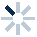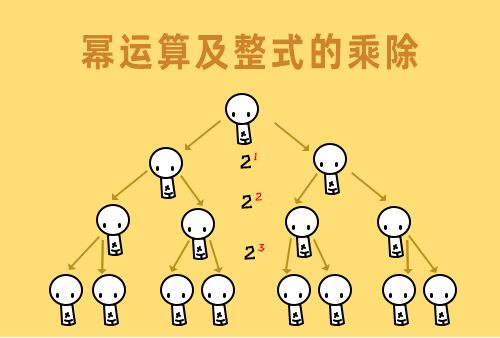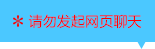| | |

||
APP端下载AndroidiPhone
|
￥48

|
2358人点赞
209311人已学习
|

3天无理由退款

3天无理由退款：退款将以超级币形式退至您的超级课堂学习账户，便于您重新选购其他课程。恶意退款将被冻结账号。

• 1、一元二次方程的特点：(1)整式方程，(2)只含有一个未知数，(3)未知数的最高次数为$2$。
2、 一元二次方程的一般形式，$a$叫做二次项系数，$b$叫做一次项系数，$c$是常数项。只有将方程化为一般形式，才有这些概念。
3、 一元二次方程最重要的特征，只需满足二次项系数$a\neq 0$，对$b$和$c$不作限制。
4、 代入法：检验数值是否为方程的根，将数值代入方程，验证方程左右两边是否相等。
• 1、最简单的直接开平方法，常见的使用情形有三种，$x^{2}=a(a\geq 0)$，$(x-a)^{2}=b(b\geq 0)$，$(x-a)^{2}=k(x-b)^{2}(k＞0)$，遇到这三种情况，直接考虑两边同时开方。但是一定要注意结果正负号的保留。
2、 因式分解法求解一元二次方程，分为三步，先把原式化为一般形式，再将等号左边的多项式分解因式。最后，根据乘法原则求出方程的根。
• 1、配方法解方程的步骤总结为一首七言绝句：二次系数化为一，常数要往右边移，一次系数一半方，有借有还讲道理。
2、 把二次项系数化为$1$后，要配的常数就是一次项系数一半的平方。
3、 加上这个常数后，你还一定要减去这个常数，或者在等式的另一边也加上这个常数，这是为了维持等式的恒等。
• 1、我们介绍了求根公式$x=\dfrac{-b\pm \sqrt{b^{2}-4ac}}{2a}$，你要熟悉它的推导过程，利用了配方法，它就是配方法处理好，留给我们的模具，只需要傻瓜级别的代入求值。
• 1、利用公式法解方程，要先把方程整理为一般形式，找出系数$a$、$b$、$c$，然后代入$\dfrac{-b\pm \sqrt{b^{2}-4ac}}{2a}$。总而言之呢，就是“公式一记牢，傻瓜也自豪”。
• 1、一元二次方程根的判别式，$\Delta =b^{2}-4ac$。当$\Delta > 2、 0$时，方程有两个不等的根。；当$\Delta =0$时，方程有两个相等的根；当$\Delta < 3、 0$时，方程没有根
4、 根据题目告诉你的根的特点，利用判别式可以确定题目中未知参数的取值范围。
5、 利用判别式证明一元二次方程根是否存在固定的情况，比如绝对有两个不相等的实数根
6、 根的判别式通常只是解题的第一步，它只能大致判断出根的性质，至于根与系数之间存在的具体关系，这种小纠纷就不在大法官的管辖内了，这时你就要用韦达定理来分析。
• 1、韦达定理的基本内容两根之和等于$a$分之$-b$，两根之积等于$a$分之$c$，以及延伸公式$\left | x_{1}-x_{2} \right |$。
2、 利用韦达定理表达出两根之间的特殊关系两根的平方和，和两根倒数之和。
3、 已知两根之和与两根之积写出原方程$x^{2}+(x_{1}+x_{2})x+x_{1}x_{2}=0$。
• 一元二次方程综合练习

• 1随风而舞
• 2喜总ya
• 3唯爱我段
• 4超级学员2672514
• 5超级学员2801917
• 6超级学员2810136
• 7超级学员3
• 8超级学员3947914
• 9超级学员4033053
• 10超级学员4049328210323人在学
￥ 88 ￥ 88260164人在学
￥ 12 ￥ 12209003人在学
￥ 88 ￥ 88209288人在学
￥ 63 ￥ 63

• 0
• 点击分享有好礼
••app端下载关注微信号